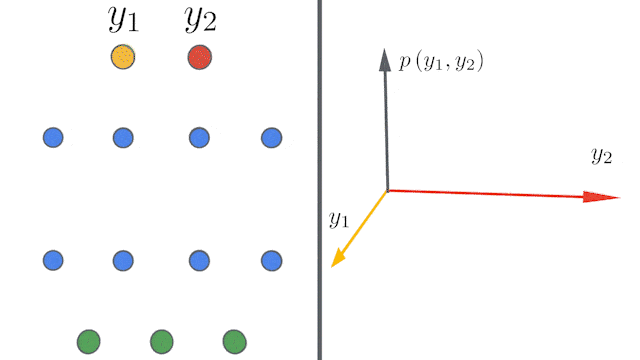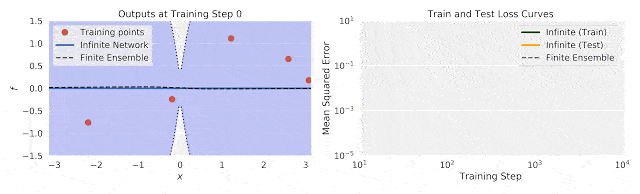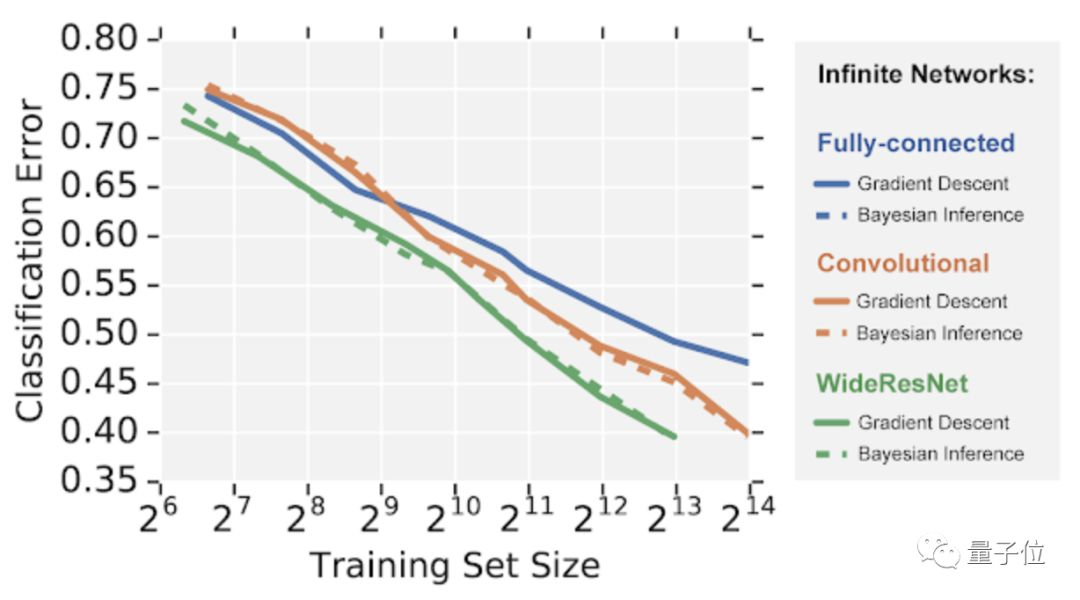# 谷歌重磅开源新技术：5行代码打造无限宽神经网络模型，帮助“打开ML黑匣子”

2020-03-14 12:35:39    创事记 微博

##### 鱼羊 假装发自 凹非寺量子位 报道 | 公众号 QbitAINeural Tangents 是一个高级神经网络 API，可用于指定复杂、分层的神经网络，在 CPU/GPU/TPU 上开箱即用。

`from neural_tangents import predict, staxinit_fn, apply_fn, kernel_fn = stax.serial(    stax.Dense(2048, W_std=1.5, b_std=0.05), stax.Erf(),    stax.Dense(2048, W_std=1.5, b_std=0.05), stax.Erf(),    stax.Dense(1, W_std=1.5, b_std=0.05))y_mean, y_var = predict.gp_inference(kernel_fn, x_train, y_train, x_test, ‘ntk’, diag_reg=1e-4, compute_cov=True)`https://arxiv.org/abs/1912.02803

GitHub地址：

Colab地址：